### Home > A2C > Chapter 6 > Lesson 6.1.3 > Problem6-49

6-49.
1. Solve each of the following equations. Homework Help ✎

1.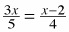2.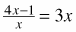3.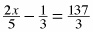4.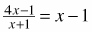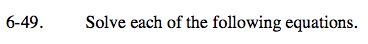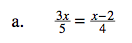$\textit{x}=-\frac{10}{7}$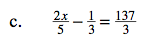x = 115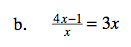4x − 1 = 3x2

3x2 − 4x + 1 = 0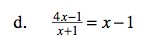Use the same method as in part (b).